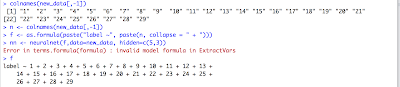#Ok, so you read a bunch of stuff on how to do Neural Networks and how many layers or nodes you should add, and etc... But when you start to implement the actual Neural Network you face a ton of dummy errors that stop your beautiful inspirational programming.

This post talks about some errors you might face when using the neuralnet package in R.

First, remember, to user the package you should install it:

install.packages("neuralnet")

Then type

library("neuralnet")

Error 1

One error that might happen training your neural network is this:

nn <- neuralnet(formula1,data=new_data, hidden=c(5,3))

Error in terms.formula(formula) : invalid model formula in ExtractVars

This happens when the name of the variables in formula "formula1" are in a non desired format. For example if you named your columns (variables) as numbers (!) you would get this error. So change your column names and re-run the model!

Exemple:

label ~ 1 + 2 + 3 + 4 + 5

Change to:

label ~ v1 + v2 + v3 + v4 + v5Error 2

Another error you might get is the following:

nn <- neuralnet(f, data=train[,-1], hidden=c(3,3))

Warning message:

algorithm did not converge in 1 of 1 repetition(s) within the stepmax

To solve this, you can increase the size of "stepmax" parameter:

nn <- neuralnet(f, data=train[,-1], hidden=c(3,3), stepmax=1e6)

If that doesn't work, you might have to change other parameters to make it converge.  Try reduce the number of hidden nodes or layers. Or changing your training data size.

Error 3

The third error I want to discuss happens when actually computing the output of the neural network:

``net.compute <- compute(net, matrix.train2[,1:10])``
``Error in neurons[[i]] %*% weights[[i]] : non-conformable arguments``

This error occurs when the number of columns in the dataframe you using to predict is different from the columns used to train the neural network. The data frames used in neuralnet and compute should have the same columns and the same names!

That is it! If you faced any other dummy error with the neuralnet package send me and I can add it to the post! Good luck! :D

Views: 7154

Comment

Join Data Science Central